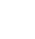# Check if cell is a number

This quick piece of code will run down the current sheet and if the value in the cell is NOT a number or is BLANK it will give you a messagebox of what the value is.

This is useful for when you want to determine if a particular cell has a number, and if not to delete that row.

``` For r = 1 To 12 If Not IsNumeric(ActiveSheet.Range("A" & r)) Or ActiveSheet.Range("A" & r) = "" Then MsgBox ActiveSheet.Range("A" & r) Next r ```